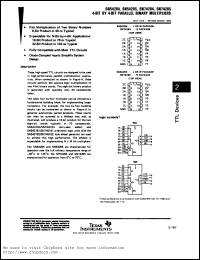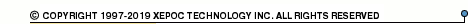More than12 555 318 queries processedSN54284 series datasheets. Manufacturer: Texas Instruments.

 SN54284J 4-bit by 4-bit parallel binary multiplier in 16-pin PDIP-T16 package. Operational temperature range from 0°C to 70°C. Datasheet*) SN54284W 4-bit by 4-bit parallel binary multiplier in 16-pin PDIP-T16 package. Operational temperature range from 0°C to 70°C. Datasheet*) SN54285J 4-bit by 4-bit parallel binary multiplier in 16-pin PDIP-T16 package. Operational temperature range from 0°C to 70°C. Datasheet*) SN54285W 4-bit by 4-bit parallel binary multiplier in 16-pin PDIP-T16 package. Operational temperature range from 0°C to 70°C. Datasheet*) SN74284N 4-bit by 4-bit parallel binary multiplier in 16-pin PDIP-T16 package. Operational temperature range from 0°C to 70°C. Datasheet*) SN74285N 4-bit by 4-bit parallel binary multiplier in 16-pin PDIP-T16 package. Operational temperature range from 0°C to 70°C. Datasheet*) SN54284 4-BIT BY 4-BIT PARALLEL BINARY MULTIPLIERS Datasheet*) SN74284 4-BIT BY 4-BIT PARALLEL BINARY MULTIPLIERS Datasheet*) SN74285 4-BIT BY 4-BIT PARALLEL BINARY MULTIPLIERS Datasheet*)